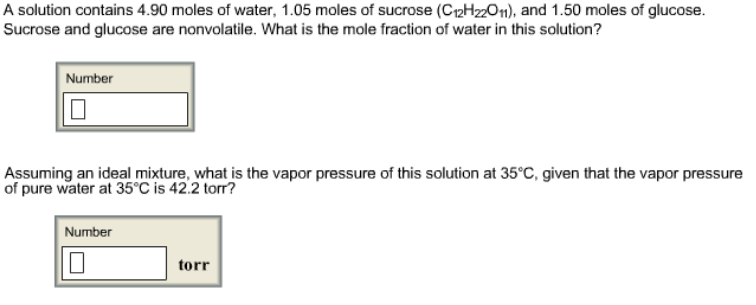# Problem: A solution contains 4.90 moles of water, 1.05 moles of sucrose (C12H22O11), and 1.50 moles of glucose. Sucrose and glucose are nonvolatile. What is the mole fraction of water in this solution? Assuming an ideal mixture, what is the vapor pressure of this solution at 35°C, given that the vapor pressure of pure water at 35°C is 42.2 torr?

###### FREE Expert Solution
87% (229 ratings)###### Problem Details

A solution contains 4.90 moles of water, 1.05 moles of sucrose (C12H22O11), and 1.50 moles of glucose. Sucrose and glucose are nonvolatile. What is the mole fraction of water in this solution?

Assuming an ideal mixture, what is the vapor pressure of this solution at 35°C, given that the vapor pressure of pure water at 35°C is 42.2 torr?